[Last time](https://forum.azimuthproject.org/discussion/2204/lecture-36-categories-from-graphs/p1) we saw that for any graph \$$G\$$ there is a free category on that graph, whose objects are nodes of \$$G\$$ and whose morphisms are paths in \$$G\$$. We can get more categories using another trick: we can add equations between paths in the graph, and still get a category! We are only allowed to equate two paths \$$f\$$ and \$$g\$$ when they are **parallel**, meaning \$$f : x \to y\$$ and \$$g: x \to y\$$ for some objects \$$x\$$ and \$$y\$$. For example, last time we saw a category coming from this graph: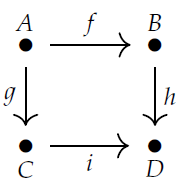This category is called the **free category on a square.** In this category there are two paths from \$$A\$$ to \$$D\$$, namely \$$h \circ f \$$ and \$$i \circ g\$$. They're not equal! But we can make up a new, smaller category where we artificially decree that \$$h \circ f = i \circ g\$$. This category is famous: it's called the **commutative square** since you can either go down and then go right to get from \$$A\$$ to \$$D\$$, or go right and then go down, and we are counting these as _the same morphism_. Both these categories are important. Imagine living on a city block with one-way roads. You live at \$$A\$$ and you are trying to visit your friend at \$$D\$$. For some purposes it may matter which route you take. Then you should use the free category on the square. But for some other purposes it may not matter - you may not care about _how_ you're going from \$$A\$$ to \$$D\$$, just _whether you can do it_. Then you should use the commutative square! Remember from [Lecture 35](https://forum.azimuthproject.org/discussion/2199/lecture-35-chapter-2-categories-versus-preorders/p1) that a preorder can be viewed as a category with at most one morphism from any object to any object. The commutative square is a nice example: in the commutative square, there's at most one morphism from any object \$$A,B,C,D\$$ to any other. We use preorders when we don't care _how_ we go from one object to another, just _whether_ you can. Indeed, we can always take _any_ category \$$\mathcal{C}\$$ and artificially throw in so many equations that we collapse it down to a preorder! This was the topic of [Puzzle 103](https://forum.azimuthproject.org/discussion/2199/lecture-35-chapter-2-categories-versus-preorders/p1). To do this, we simply say that whenever we have _any_ morphisms \$$f, g : x \to y\$$, where \$$x\$$ and \$$y\$$ are _any_ objects in our category, we decree \$$f = g\$$. The result is called the **preorder reflection of \$$\mathcal{C}\$$**. Fong and Spivak discuss this in Section 3.2.3, and they make a good point: the free category on a graph is very different from a preorder. The free category on a graph has the _fewest possible_ equations between parallel morphisms, while a preorder has the _most possible._ Anyway, our trick of building a category by first taking the free category on a graph and then imposing some equations between parallel morphisms is called **presenting** a category. The graph, together with the equations, is called a **presentation** of the category. If we use a finite graph and finitely many equations, we get a **finitely presented category**. These will be important for databases. For example, consider this graph:It has 3 nodes: Employee, Department and String. It has 5 edges: WorksIn, Secretary, DepartmentName, FirstName and Manager. If you think about it, you'll see what's going on. Every employee has a first name, which is a string of characters, and that's why we have an edge FirstName from Employee to String. In the free category on our graph, this edge gives a morphism $\textrm{FirstName} : \textrm{Employee} \to \textrm{String}.$ Similarly for the other edges. Every department has a secretary, which is an employee, and so on. We also get composite morphisms like $\textrm{FirstName} \circ \textrm{Manager} \circ \textrm{Manager} : \textrm{Employee} \to \textrm{String}$ This says that the first name of the manager of the manager of some employee is a string! We'll see next time what this stuff is good for. But we don't really want to use the free category on this graph, because there could be some interesting _equations between morphisms_. **Puzzle 106.** What are some reasonable equations between morphisms that we might want to impose? We'll see what we can do with the resulting finitely presented category! Or you can "cheat" and read the book. If you do, I should warn you that while I've been writing \$$g \circ f: x \to z\$$ for the composite of morphisms \$$f: x \to y\$$ and \$$g : y \to z\$$, Fong and Spivak write \$$f.g : x \to z\$$. Both conventions are useful. That's enough lecturing for today! I'll leave you with two more puzzles. **Puzzle 107.** Take the free category on this graph: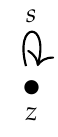and then impose the equation \$$s \circ s \circ s \circ s = 1_z\$$. You get a category with one object, also known as a **[monoid](https://en.wikipedia.org/wiki/Monoid)**. How many morphisms does this category have? How is it related to the picture below?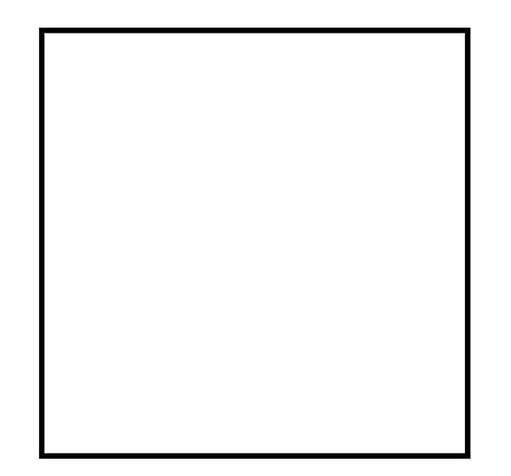**Puzzle 108.** Take the free category on this graph: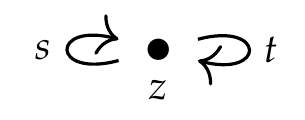and then impose the equations $s \circ s \circ s \circ s = t \circ t = s \circ t \circ s \circ t = 1_z .$ Again you get a monoid. How is it related to the picture below?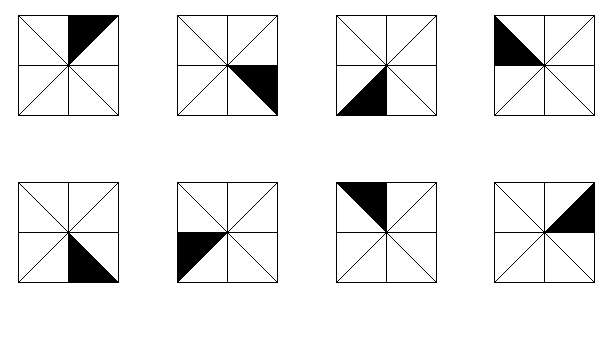These two puzzles are connected to [Puzzles 99 and 100](https://forum.azimuthproject.org/discussion/2198/lecture-34-chapter-3-categories/p1). Think about it! **[To read other lectures go here.](http://www.azimuthproject.org/azimuth/show/Applied+Category+Theory#Chapter_3)**